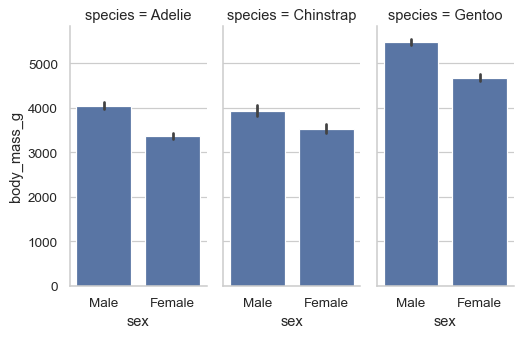# seaborn.barplot#

seaborn.barplot(data=None, *, x=None, y=None, hue=None, order=None, hue_order=None, estimator='mean', errorbar=('ci', 95), n_boot=1000, units=None, seed=None, orient=None, color=None, palette=None, saturation=0.75, fill=True, hue_norm=None, width=0.8, dodge='auto', gap=0, log_scale=None, native_scale=False, formatter=None, legend='auto', capsize=0, err_kws=None, ci=<deprecated>, errcolor=<deprecated>, errwidth=<deprecated>, ax=None, **kwargs)#

Show point estimates and errors as rectangular bars.

A bar plot represents an aggregate or statistical estimate for a numeric variable with the height of each rectangle and indicates the uncertainty around that estimate using an error bar. Bar plots include 0 in the axis range, and they are a good choice when 0 is a meaningful value for the variable to take.

Note

By default, this function treats one of the variables as categorical and draws data at ordinal positions (0, 1, … n) on the relevant axis. As of version 0.13.0, this can be disabled by setting `native_scale=True`.

Parameters:
dataDataFrame, Series, dict, array, or list of arrays

Dataset for plotting. If `x` and `y` are absent, this is interpreted as wide-form. Otherwise it is expected to be long-form.

x, y, huenames of variables in `data` or vector data

Inputs for plotting long-form data. See examples for interpretation.

order, hue_orderlists of strings

Order to plot the categorical levels in; otherwise the levels are inferred from the data objects.

estimatorstring or callable that maps vector -> scalar

Statistical function to estimate within each categorical bin.

errorbarstring, (string, number) tuple, callable or None

Name of errorbar method (either “ci”, “pi”, “se”, or “sd”), or a tuple with a method name and a level parameter, or a function that maps from a vector to a (min, max) interval, or None to hide errorbar.

New in version v0.12.0.

n_bootint

Number of bootstrap samples used to compute confidence intervals.

unitsname of variable in `data` or vector data

Identifier of sampling units; used by the errorbar function to perform a multilevel bootstrap and account for repeated measures

seedint, `numpy.random.Generator`, or `numpy.random.RandomState`

Seed or random number generator for reproducible bootstrapping.

orient“v” | “h” | “x” | “y”

Orientation of the plot (vertical or horizontal). This is usually inferred based on the type of the input variables, but it can be used to resolve ambiguity when both `x` and `y` are numeric or when plotting wide-form data.

Changed in version v0.13.0: Added ‘x’/’y’ as options, equivalent to ‘v’/’h’.

colormatplotlib color

Single color for the elements in the plot.

palettepalette name, list, or dict

Colors to use for the different levels of the `hue` variable. Should be something that can be interpreted by `color_palette()`, or a dictionary mapping hue levels to matplotlib colors.

saturationfloat

Proportion of the original saturation to draw fill colors in. Large patches often look better with desaturated colors, but set this to `1` if you want the colors to perfectly match the input values.

fillbool

If True, use a solid patch. Otherwise, draw as line art.

New in version v0.13.0.

hue_normtuple or `matplotlib.colors.Normalize` object

Normalization in data units for colormap applied to the `hue` variable when it is numeric. Not relevant if `hue` is categorical.

New in version v0.12.0.

widthfloat

Width allotted to each element on the orient axis. When `native_scale=True`, it is relative to the minimum distance between two values in the native scale.

dodge“auto” or bool

When hue mapping is used, whether elements should be narrowed and shifted along the orient axis to eliminate overlap. If `"auto"`, set to `True` when the orient variable is crossed with the categorical variable or `False` otherwise.

Changed in version 0.13.0: Added `"auto"` mode as a new default.

gapfloat

Shrink on the orient axis by this factor to add a gap between dodged elements.

New in version 0.13.0.

log_scalebool or number, or pair of bools or numbers

Set axis scale(s) to log. A single value sets the data axis for any numeric axes in the plot. A pair of values sets each axis independently. Numeric values are interpreted as the desired base (default 10). When `None` or `False`, seaborn defers to the existing Axes scale.

New in version v0.13.0.

native_scalebool

When True, numeric or datetime values on the categorical axis will maintain their original scaling rather than being converted to fixed indices.

New in version v0.13.0.

formattercallable

Function for converting categorical data into strings. Affects both grouping and tick labels.

New in version v0.13.0.

legend“auto”, “brief”, “full”, or False

How to draw the legend. If “brief”, numeric `hue` and `size` variables will be represented with a sample of evenly spaced values. If “full”, every group will get an entry in the legend. If “auto”, choose between brief or full representation based on number of levels. If `False`, no legend data is added and no legend is drawn.

New in version v0.13.0.

capsizefloat

Width of the “caps” on error bars, relative to bar spacing.

err_kwsdict

Parameters of `matplotlib.lines.Line2D`, for the error bar artists.

New in version v0.13.0.

cifloat

Level of the confidence interval to show, in [0, 100].

Deprecated since version v0.12.0: Use `errorbar=("ci", ...)`.

errcolormatplotlib color

Color used for the error bar lines.

Deprecated since version 0.13.0: Use `err_kws={'color': ...}`.

errwidthfloat

Thickness of error bar lines (and caps), in points.

Deprecated since version 0.13.0: Use `err_kws={'linewidth': ...}`.

axmatplotlib Axes

Axes object to draw the plot onto, otherwise uses the current Axes.

kwargskey, value mappings

Other parameters are passed through to `matplotlib.patches.Rectangle`.

Returns:
axmatplotlib Axes

Returns the Axes object with the plot drawn onto it.

`countplot`

Show the counts of observations in each categorical bin.

`pointplot`

Show point estimates and confidence intervals using dots.

`catplot`

Combine a categorical plot with a `FacetGrid`.

Notes

For datasets where 0 is not a meaningful value, a `pointplot()` will allow you to focus on differences between levels of one or more categorical variables.

It is also important to keep in mind that a bar plot shows only the mean (or other aggregate) value, but it is often more informative to show the distribution of values at each level of the categorical variables. In those cases, approaches such as a `boxplot()` or `violinplot()` may be more appropriate.

Examples

With long data, assign `x` and `y` to group by a categorical varaible and plot aggregated values, with confidence intervals:

```sns.barplot(penguins, x="island", y="body_mass_g")
```Prior to v0.13.0, each bar would have a different color. To replicate this behavior, assign the grouping variable to `hue` as well:

```sns.barplot(penguins, x="body_mass_g", y="island", hue="island", legend=False)
```When plotting a “wide-form” dataframe, each column will be aggregated and represented as a bar:

```flights_wide = flights.pivot(index="year", columns="month", values="passengers")
sns.barplot(flights_wide)
```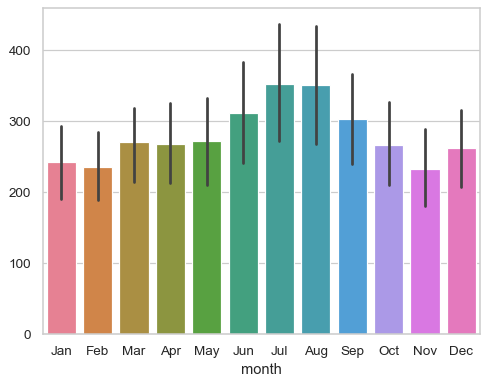Passing only a series (or dict) will plot each of its values, using the index (or keys) to label the bars:

```sns.barplot(flights_wide["Jun"])
```With long-form data, you can add a second layer of grouping with `hue`:

```sns.barplot(penguins, x="island", y="body_mass_g", hue="sex")
```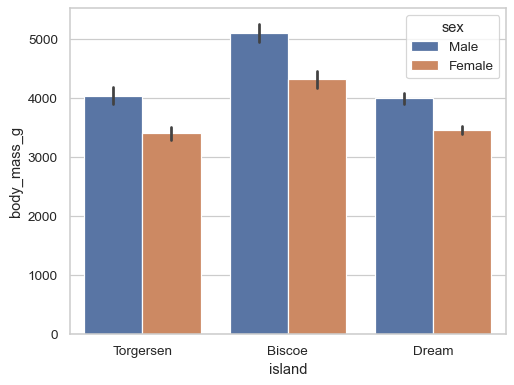Use the error bars to show the standard deviation rather than a confidence interval:

```sns.barplot(penguins, x="island", y="body_mass_g", errorbar="sd")
```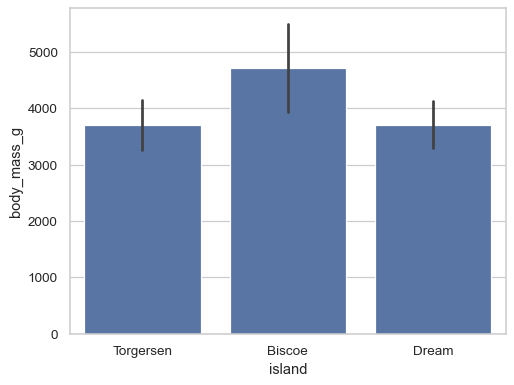Use a different aggregation function and disable the error bars:

```sns.barplot(flights, x="year", y="passengers", estimator="sum", errorbar=None)
```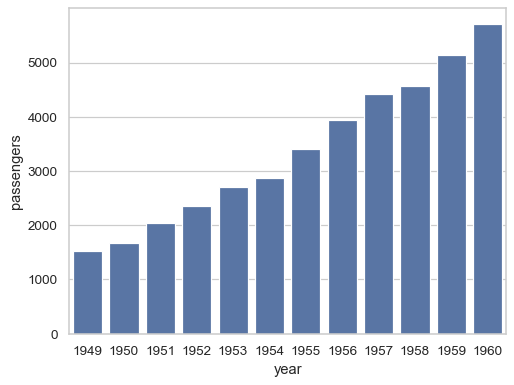Add text labels with each bar’s value:

```ax = sns.barplot(flights, x="year", y="passengers", estimator="sum", errorbar=None)
ax.bar_label(ax.containers, fontsize=10);
```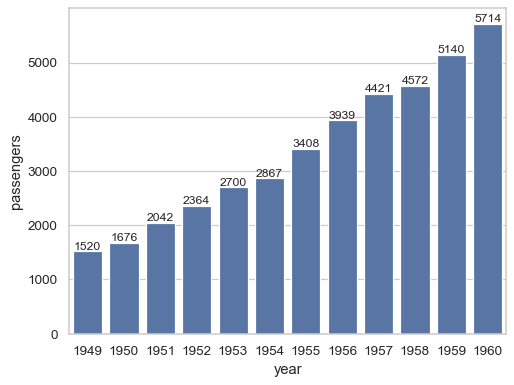Preserve the original scaling of the grouping variable and add annotations in numeric coordinates:

```ax = sns.barplot(
flights, x="year", y="passengers",
native_scale=True,
estimator="sum", errorbar=None,
)
ax.plot(1955, 3600, "*", markersize=10, color="r")
```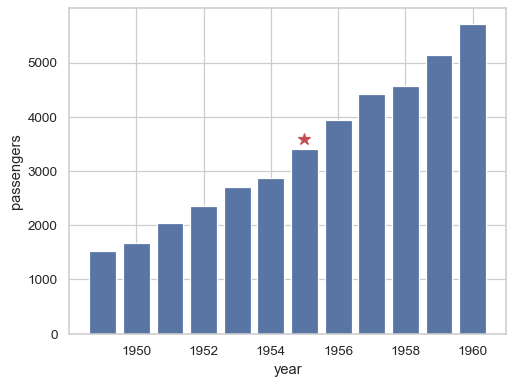Use `orient` to resolve ambiguity about which variable should group when both are numeric:

```sns.barplot(flights, x="passengers", y="year", orient="y")
```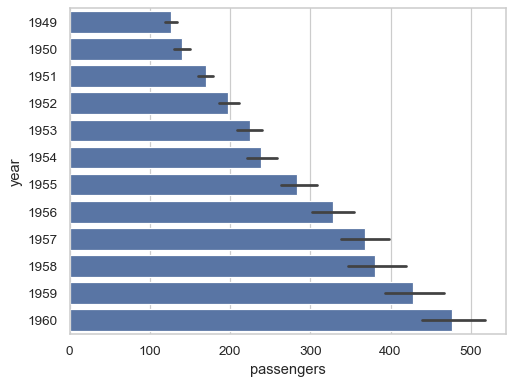Customize the appearance of the plot using `matplotlib.patches.Rectangle` and `matplotlib.lines.Line2D` keyword arguments:

```sns.barplot(
penguins, x="body_mass_g", y="island",
errorbar=("pi", 50), capsize=.4,
err_kws={"color": ".5", "linewidth": 2.5},
linewidth=2.5, edgecolor=".5", facecolor=(0, 0, 0, 0),
)
```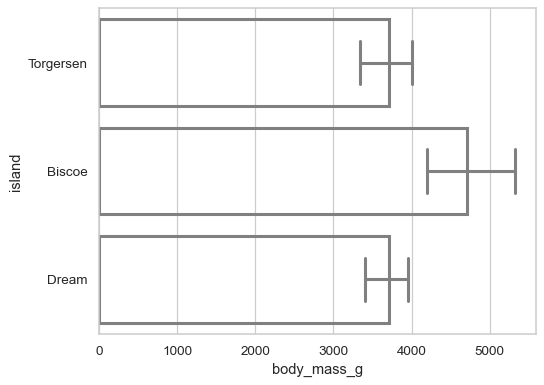Use `catplot()` to draw faceted bars, which is recommended over working directly with `FacetGrid`:

```sns.catplot(
penguins, kind="bar",
x="sex", y="body_mass_g", col="species",
height=4, aspect=.5,
)
```